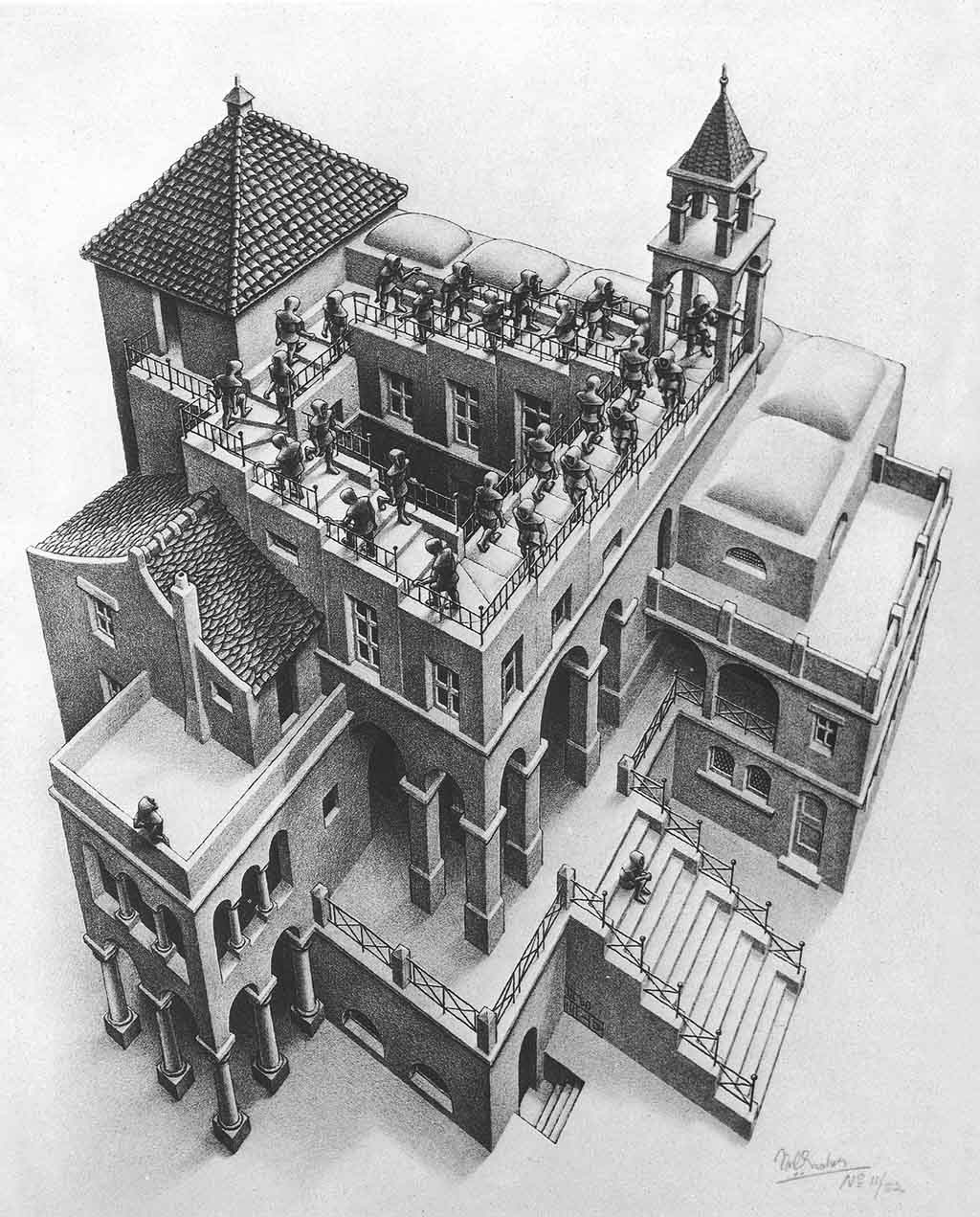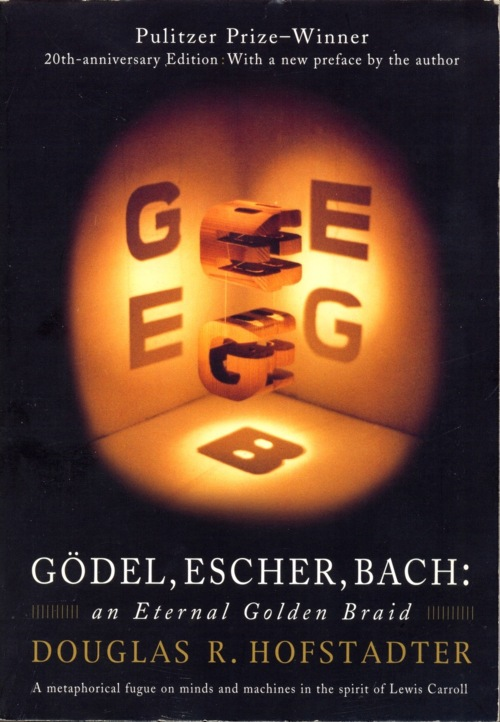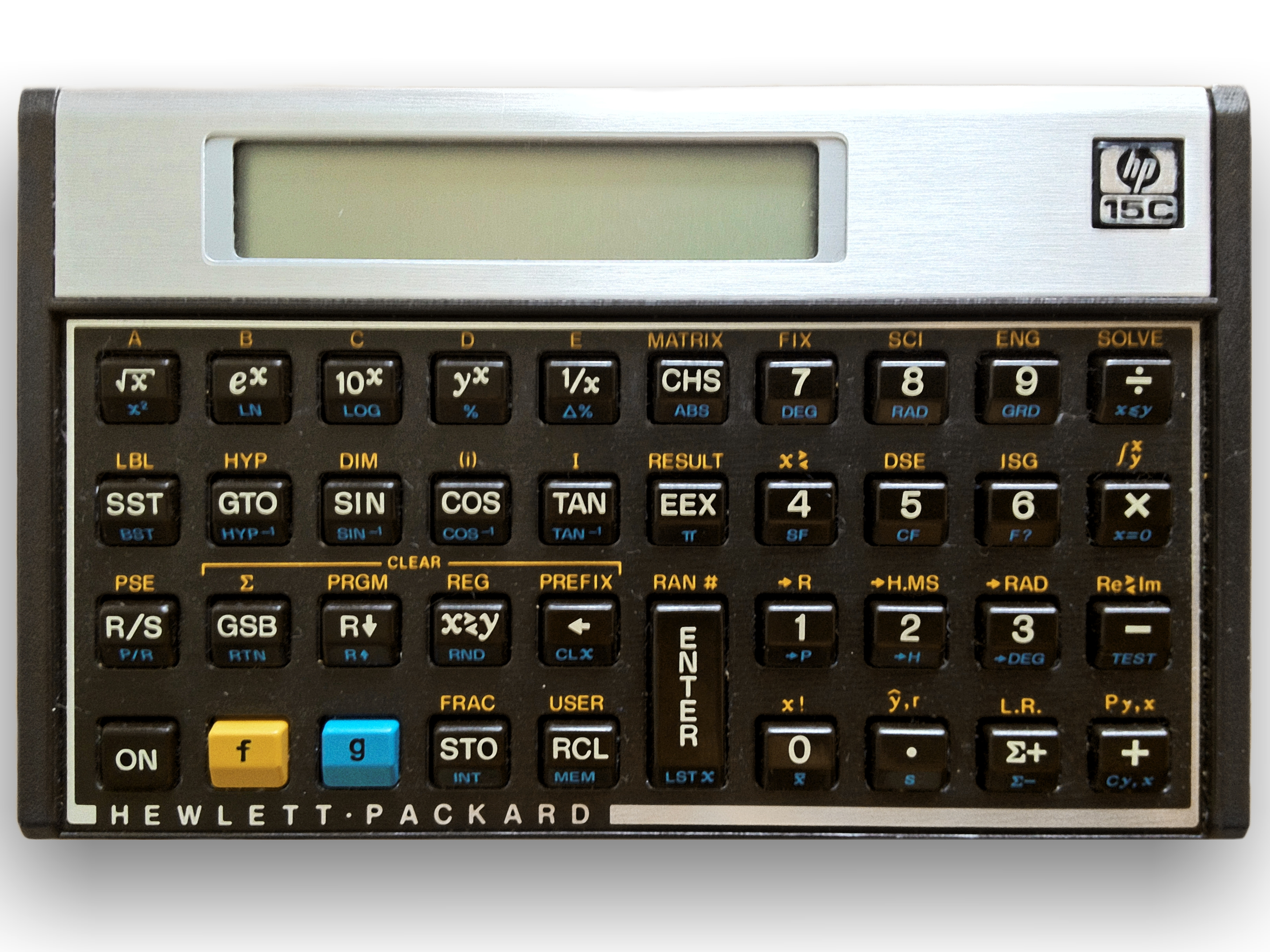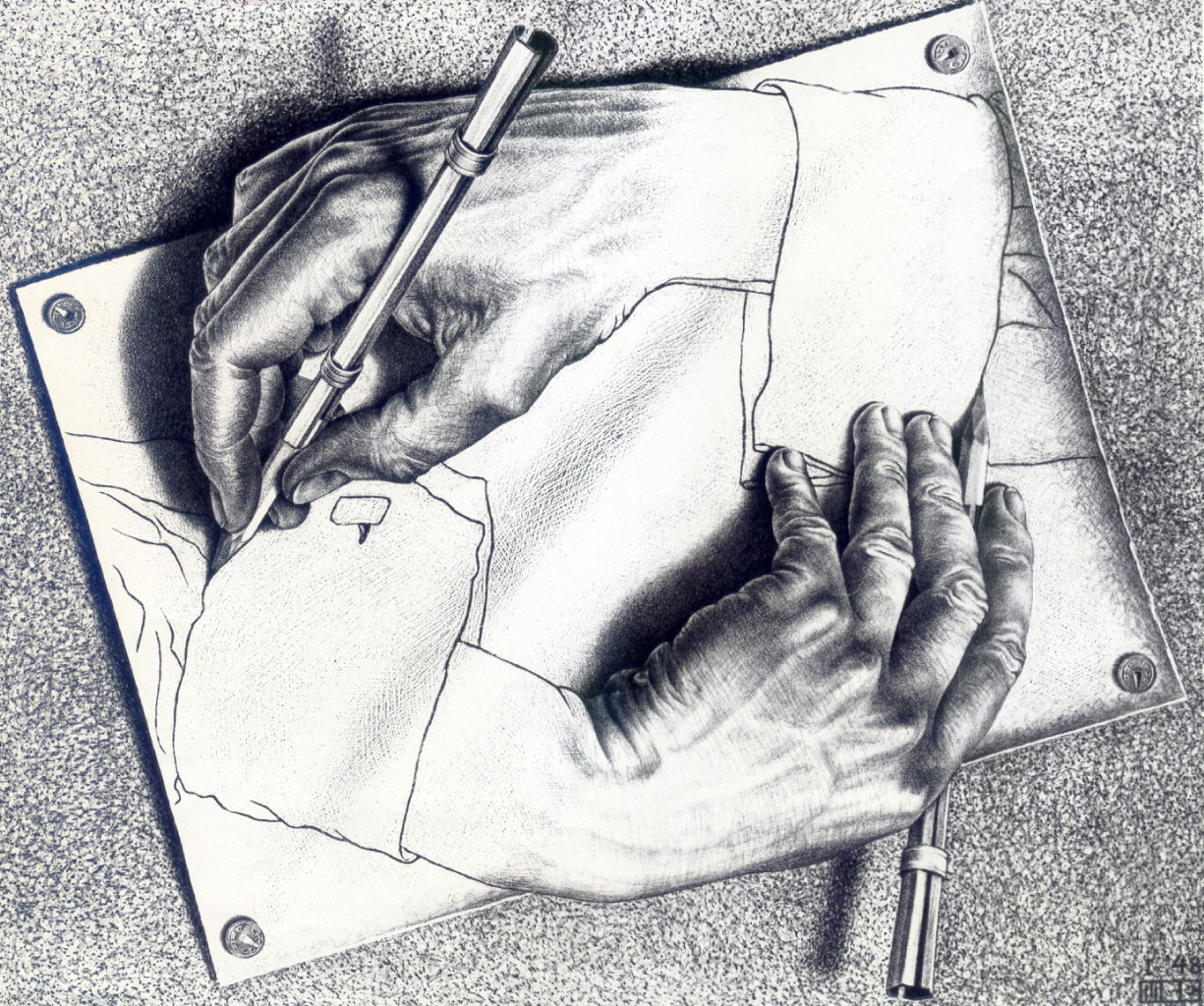# Why Y?

## Why Y?## Fixed point## Cosine

```			`-1`
`cos(-1) -> 0.540302...`
`cos(cos(-1)) -> 0.857553...`
`...`
`cos(cos(...cos(-1)...)) -> 0.739085...`
```

## Fixed point

``x = f(x) = f(f(x)) = f(f(f(x))) = ...``
```			`f(x) = cos(x) -> fixpoint = 0.739085...`
`f(x) = x2 - 3x + 4 -> fixpoint = 2`
`f(x) = x2 -> fixpoint = 0, fixpoint = 1`
`f(x) = x + 1 -> no fixpoint`
```

## Y combinator

Tiger got to hunt,
Bird got to fly;
Lisper got to sit and wonder, (Y (Y Y))?

Tiger got to sleep,
Bird got to land;
Lisper got to tell himself he understand.

— Kurt Vonnegut, modified by Darius Bacon

## Factorial

```				`function commonFactorial(n) {`
`	return n === 0 ? 1 : n * commonFactorial(n-1);`
`}`
```

## Non-recursive factorial

```				`function fact(self) {`
`	return function(n) {`
`		return n === 0 ? 1 : n * self(n-1);`
`	};`
`}`
```

## Notation

```			`function fact(self)(n) {`
`	return n === 0 ? 1 : n * self(n-1);`
`}`
```

## Fixed point

``commonFactorial``
is a fixed point of
``fact``
!

```				`fact(commonFactorial)(4) === commonFactorial(4);`
`fact(commonFactorial)(10) === commonFactorial(10);`
`fact(commonFactorial)(17) === commonFactorial(17);`
`...`
`//But we are trying to calculate factorial without recursion!`
```

## Y comes to help!

What if there exists function
``Y``
that we could pass
``fact``
to that would return the fixed point of it, i.e.
``commonFactorial``
function?

```			`Y(fact)(42)`
` === commonFactorial(42)`
` === fact(commonFactorial)(42)`
` === fact(Y(fact))(42)`
`//So we do not need commonFactorial function at all!`
```

## Fixed point combinator

```			`function fix(f) {`
`	var p = function(self)(n) {`
`		return f(self(self))(n);`
`	};`
`	return p(p);`
`}`
`var factorial = fix(fact);`
`factorial(6); // => 720`
```

## Wrappers## Simple logging wrapper

```			`function logWrapper(f)(self)(n) {`
`	var result = f(self)(n);`
`	console.log('f(' + n + ') = ' + result);`
`	return result;`
`}`
`var logFactorial = fix(logWrapper(fact));`
`logFactorial(3); // => 6`
```

## Trampoline## Tail call optimization

```			`function commonFactorial(n, r) {`
`	return n === 0 ? r : commonFactorial(n - 1, n*r);`
`};`
```
```			`function fact(self)(n, r) {`
`	return n === 0 ? r : self(n - 1, n*r);`
`}`
```

## Trampoline

```			`function fix(f)(n, r) {`
`	var a = function(n_, r_) {`
`		n = n_; r = r_;`
`		return a;`
`	};`
`	while (a && instanceof Function) { a = f(a)(n, r); }`
`	return a;`
`}`
`var factorial = fix(fact);`
```

## Quine

A quine is a program which prints its own listing.

```				`(function \$(){console.log('('+ \$ +'());')}());`
```

## Principle

Program consists of two parts, one which call the code and one which we call the data.

 SAY "SAY" verb noun code data

## Quine

```				`var newLine = String.fromCharCode(10);`
`//intron`
`var data = 'var newLine = String.fromCharCode(10);%3//intron%3var data = %1%0%1;%3console.log(data.replace(/%21/g, "%1").replace(/%23/g, newLine).replace(/%22/g, "%").replace("%20", data));';`
`console.log(data.replace(/%1/g, "'").replace(/%3/g, newLine).replace(/%2/g, "%").replace("%0", data));`
```

## Tupper's formulawhen graphed in two dimensions, can visually reproduce itself## Tupper's formula